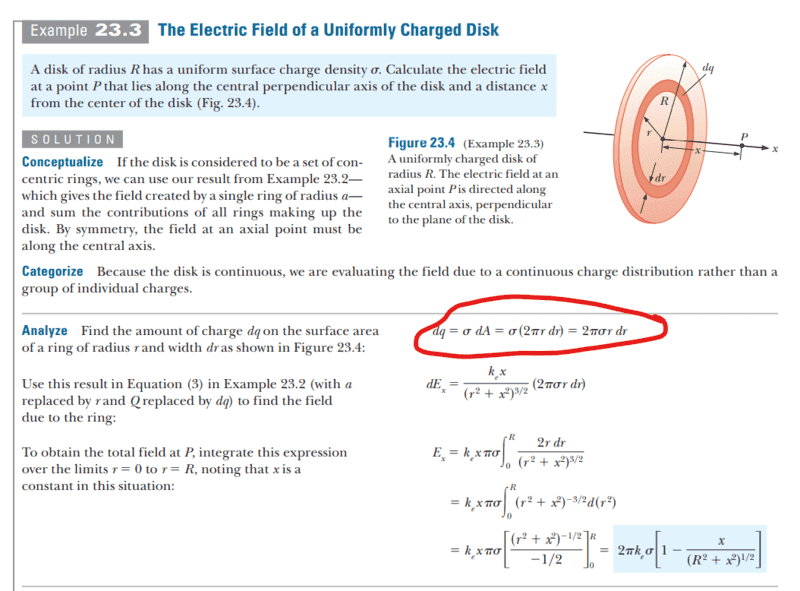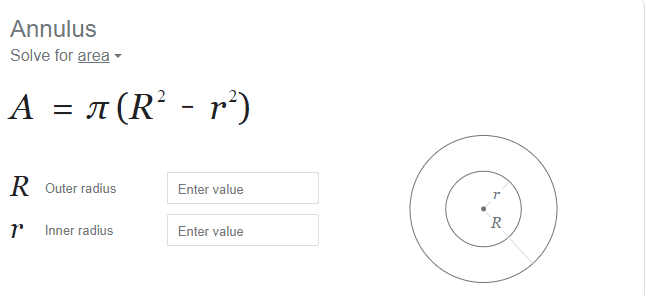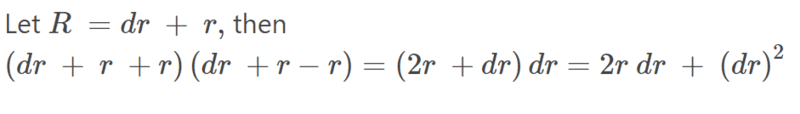# Finding Area of Ring Segment to Find Electric Field of Disk

Callumnc1
Homework Statement:
A disk of radius R has a uniform surface charge density s. Calculate the electric field at a point P that lies along the central perpendicular axis of the disk and a distance x from the center of the disk
Relevant Equations:
Continuous charge distribution formula
Hi!

For this problem,Why is the area of each ring segment dA equal to (2π)(r)(dr)?

However, according to google the area of a ring segment (Annulus) is,Many thanks!

Homework Helper
Gold Member
Cut the thin ring and stretch it out. You get a rectangular strip of length ##2\pi r## and width ##dr##. What is its area?

The area of the annulus is indeed what google says. Note that
##R^2-r^2=(R+r)(R-r)##
For a thin ring ##R## and ##r## are very close to each other so that ##R-r=dr##. You can then approximate ##R+r\approx 2r## so that ##R^2-r^2\approx 2rdr##.

•Callumnc1
Callumnc1
Cut the thin ring and stretch it out. You get a rectangular strip of length ##2\pi r## and width ##dr##. What is its area?
Thanks! So that is thought process that gives the area! However, why is that not equal to the area from the Annulus formula?

From the annulus formula I got A = (π)[(r + dr)^2 - r^2].

Many thanks!

Homework Helper
Gold Member
Thanks! So that is thought process that gives the area! However, why is that not equal to the area from the Annulus formula?

From the annulus formula I got A = (π)[(r + dr)^2 - r^2].

Many thanks!
See my edited post.

•Callumnc1
Callumnc1
See my edited post.
Thank you, I have written up and realized I had a new question:What if we choose not to approximate? How would we integrate the infinitesimal (dr)^2 term?

Many thanks!

Gold Member
Thank you, I have written up and realized I had a new question:
View attachment 318038
What if we choose not to approximate? How would we integrate the infinitesimal (dr)^2 term?

Many thanks!
Well, you will notice that if you integrate

$$\int dA = 2\pi \int_{r}^{R} r~dr$$

That it is exactly:

$$A = \pi \left( R^2 - r^2 \right)$$

So the term ##(dr)^2## is not contributing anything. In the limit as ##dr \to 0 , 2 r ~dr ## is exact.

•Callumnc1
Callumnc1
Well, you will notice that if you integrate

$$\int dA = 2\pi \int_{r}^{R} r~dr$$

That it is exactly:

$$A = \pi \left( R^2 - r^2 \right)$$

So the term ##(dr)^2## is not contributing anything. In the limit as ##dr \to 0 , 2 r ~dr ## is exact.

I guess as dr approaches zero then (dr)^2 approach's zero a lot faster than 2r dr because it is squared.

However, just curious had would you integrate the (dr)^2 term anyway?

Many thanks!

Gold Member
However, just curious had would you integrate the (dr)^2 term anyway?
I don't believe you ever would. It doesn't follow from the definition of the derivative.

$$(A + \Delta A)- A = \pi \left[ \left( r + \Delta r \right)^2 - r^2\right] \implies \Delta A = \pi \left[ 2 r ~\Delta r + (\Delta r)^2 \right]$$

The derivative is given by:

$$\frac{dA}{dr} = \lim_{ \Delta r \to 0 } \frac{\Delta A}{ \Delta r} = \lim_{ \Delta r \to 0 } \frac{ \pi \left[ 2 r ~\Delta r + (\Delta r)^2 \right] }{ \Delta r} = \lim_{ \Delta r \to 0 } \pi [ 2r + \cancel{ (\Delta r)}^0 ] = 2 \pi r$$

So if there are any first order ( or higher ) ##\Delta r ## terms left hanging around they vanish in the limit.

Last edited:
•Callumnc1
Callumnc1
I don't believe you ever would. It doesn't follow from the definition of the derivative.

$$(A + \Delta A)- A = \pi \left[ \left( r + \Delta r \right)^2 - r^2\right] \implies \Delta A = \pi \left[ 2 r ~\Delta r + (\Delta r)^2 \right]$$

The derivative is given by:

$$\frac{dA}{dr} = \lim_{ \Delta r \to 0 } \frac{\Delta A}{ \Delta r} = \lim_{ \Delta r \to 0 } \frac{ \pi \left[ 2 r ~\Delta r + (\Delta r)^2 \right] }{ \Delta r} = \lim_{ \Delta r \to 0 } \pi [ 2r + \cancel{ (\Delta r)}^0 ] = 2 \pi r$$
Ok thank you erobz!

•erobz
Homework Helper
If you don't want to see these higher order terms in dr or ##\Delta r##, you can just start from the area of a circle, ##A(r)=\pi r^2## as a function of r. Then the change in the area when the radius increases by dr is the differential ##dA= (\frac{dA}{dr}) dr## = ##2\pi r dr##. This is the area of the ring added to the circle by a change in radius of dr so it is the area of a differential ring. You can use the same method to find the volume of a spherical shell by starting with the volume of a sphere.

•Callumnc1
Callumnc1
If you don't want to see these higher order terms in dr or ##\Delta r##, you can just start from the area of a circle, ##A(r)=\pi r^2## as a function of r. Then the change in the area when the radius increases by dr is the differential ##dA= (\frac{dA}{dr}) dr## = ##2\pi r dr##. This is the area of the ring added to the circle by a change in radius of dr so it is the area of a differential ring. You can use the same method to find the volume of a spherical shell by starting with the volume of a sphere.
Thank you for sharing with me that really interesting method @nasu !